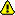[Documentation] [TitleIndex] [WordIndex]

Contentswork in progress, not actually used. The representation of robot configurations for particular groups of joints can be also specified using srdf

<model_state> element

The state element describes the basic state of a corresponding URDF model.

Here is an example of a model state element:

<model_state model="pr2" time_stamp="0.1">
<joint_state joint="r_shoulder_pan_joint" position="0" velocity="0" effort="0"/>
<joint_state joint="r_shoulder_lift_joint" position="0" velocity="0" effort="0"/>
</model_state>

Model State

<model_state>

• model (required) (string)

• The name of the model in corresponding URDF.
• time_stamp (optional) (float) (sec)

• Time stamp of this state in seconds.
• <joint_state> (optional) (string)

• joint (required) (string)

• The name of the joint this state refers to.
• position (optional) (float or array of floats)

• position for each degree of freedom of this joint
• velocity (optional) (float or array of floats)

• velocity for each degree of freedom of this joint
• effort (optional) (float or array of floats)

• effort for each degree of freedom of this joint

2019-10-12 13:15